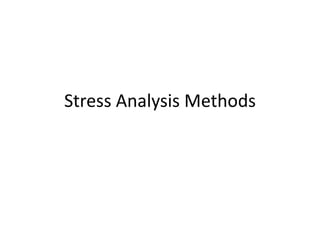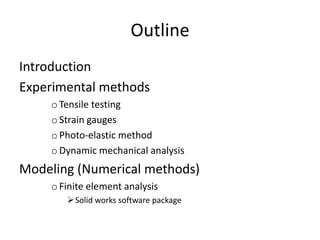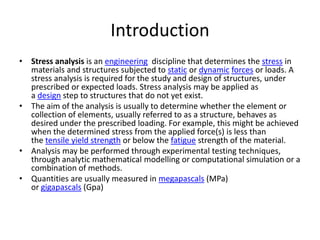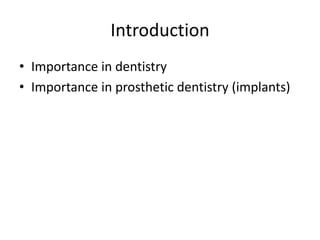Anzeige1 von 4
Anzeige

### Stress analysis methods

1. Stress Analysis Methods
2. Outline Introduction Experimental methods o Tensile testing o Strain gauges o Photo-elastic method o Dynamic mechanical analysis Modeling (Numerical methods) o Finite element analysis  Solid works software package
3. Introduction • Stress analysis is an engineering discipline that determines the stress in materials and structures subjected to static or dynamic forces or loads. A stress analysis is required for the study and design of structures, under prescribed or expected loads. Stress analysis may be applied as a design step to structures that do not yet exist. • The aim of the analysis is usually to determine whether the element or collection of elements, usually referred to as a structure, behaves as desired under the prescribed loading. For example, this might be achieved when the determined stress from the applied force(s) is less than the tensile yield strength or below the fatigue strength of the material. • Analysis may be performed through experimental testing techniques, through analytic mathematical modelling or computational simulation or a combination of methods. • Quantities are usually measured in megapascals (MPa) or gigapascals (Gpa)
4. Introduction • Importance in dentistry • Importance in prosthetic dentistry (implants)
Anzeige